### Home > CAAC > Chapter 14 > Lesson 14.2.1.1 > Problem2-8

2-8.
1. For the function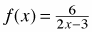, find the value of each expression below. Homework Help ✎

1. f (1)

2. f (0)

3. f (−3)

4. f (1.5)

5. What value of x would make f(x) = 4?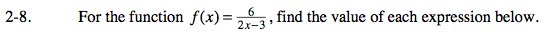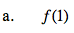Substitute 1 for x.

f(1) = −6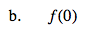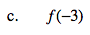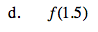f(1.5) = Undefined. You can't divide by 0.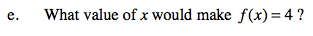Substitute 4 for f(x).

x = 2.25